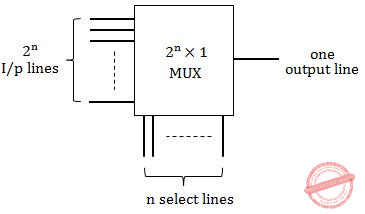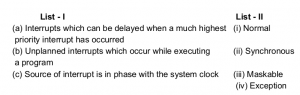## Match-the-following

 Question 1

Match the following in Software Engineering :

```        List-I                            List-II
(a) Product Complexity          (i) Software Requirements Definition
(b) Structured System Analysis (ii) Software Design
(c) Coupling and Cohesion     (iii) Validation Technique
(d) Symbolic Execution         (iv) Software Cost Estimation

```
 A (a)-(ii), (b)-(iii), (c)-(iv), (d)-(i) B (a)-(iii), (b)- (i), (c)-(iv), (d)-(ii) C (a)-(iv), (b)- (i), (c)-(ii), (d)-(iii) D (a)-(iii), (b)- (iv), (c)-(i), (d)-(ii)
Software-Engineering       Match-the-following       UGC-NET JUNE Paper-2
Question 1 Explanation:
→ Coupling and Cohesion are used in software design. Cohesion measures strength of a module while coupling measures interdependency between modules.
→ Software cost estimation must be done more diligently throughout the project life cycle so that in the future there are fewer surprises and unforeseen delays in the release of a product.
→ Validation Technique → Symbolic Execution
→ Software Requirements Definition → Structured System Analysis
 Question 2

Which of the following statements are true ?

(a) Three broad categories of Networks are
(i) Circuit Switched Networks
(ii) Packet Switched Networks
(iii) Message Switched Networks
(b) Circuit Switched Network resources need not be reserved during the set up phase.
(c) In packet switching there is no resource allocation for packets.
Code :
 A (a) and (b) only B (b) and (c) only C (a) and (c) only D (a), (b) and (c)
Computer-Networks       Match-the-following       UGC-NET JUNE Paper-2
Question 2 Explanation:
→ There are three broad categories of Networks Circuit Switched Networks, Packet Switched Networks, Message Switched Networks.
→ In circuit switching resources are reserved from Setup phase to data transmission phase.
→ When data transmission completes only then the reserved resources are released.
→ Since in packet switching each packet follows different path to reach the destination so no resource reservation is done in case of packet switching.
 Question 3

Match the terms in List-I with the options given in List-II :

```    List-I                      List-II
(a) Decoder             (i) 1 line to 2n lines
(b) Multiplexer        (ii) n lines to 2n lines
(c) De multiplexer    (iii) 2n lines to 1 line
(iv) 2n lines to 2n-1 lines

```
 A (a)-(ii), (b)-(i), (c)-(iii) B (a)-(ii), (b)-(iii), (c)-(i) C (a)-(ii), (b)-(i), (c)-(iv) D (a)-(iv), (b)-(ii), (c)-(i)
Computer-Organization       Match-the-following       UGC-NET JUNE Paper-2
Question 3 Explanation:
Decoder:Multiplexer: Many to OneDemultiplexer: One to ManyQuestion 4

Match the items in List-I and List-II :

```                      List-I                                                 List-II
(a) Interrupts which can be delayed when a much highest      (i) Normal priority interrupt has occurred
(b) Unplanned interrupts which occur while executing        (ii) Synchronous a program
(c) Source of interrupt is in phase with the system clock  (iii) Maskable
(iv) Exception ```
 A (a)-(ii), (b)-(i), (b)-(iv) B (a)-(ii), (b)-(iv), (b)-(iii) C (a)-(iii), (b)-(i), (b)-(ii) D (a)-(iii), (b)-(iv), (b)-(ii)
Computer-Organization       Match-the-following       UGC-NET JUNE Paper-2
Question 4 Explanation:
Maskable Interrupts are those which can be masked/delayed when a higher priority interrupt occurs.
Exception :
Exception are the unplanned interrupts that occur while executing a program. Divide by zero is an example of Exception.
Synchronous:
Synchronous clocks will regularly synchronize their time with a master clock. The connection can be direct (wired), or over the air (radio, wifi or similar). The connections can be at a regular intervals, on demand or a mix.
 Question 5

Match List-1 with List-2 and choose the correct answer from the code given below

```           List 1					   List 2
(a) Greedy Best-first Search         	 (i) Selects a node for expansion if optimal path to
that node has been found
(b) A* Search	          		(ii) Avoids substantial overhead associated with
keeping the sorted queue of nodes
(c) Recursive Best-First Search        (iii) Suffers from excessive node generation
(d) Iterative-deepening A* Search   	(iv) Time complexity depends upon the quality of
heuristic```
Code:
 A (a)-(iv), (b)-(iii), (c)-(ii), (d)-(i) B (a)-(i), (b)-(iv), (c)-(iii), (d)-(ii) C (a)-(iv), (b)-(i), (c)-(ii), (d)-(iii) D (a)-(i), (b)-(ii), (c)-(iii), (d)-(iv)
Algorithms       Match-the-following       UGC-NET DEC Paper-2
Question 5 Explanation:
Greedy Best-first Search: Selects a node for expansion if optimal path to that node has been found. Best-first search is a search algorithm which explores a graph by expanding the most promising node chosen according to a specified rule. Best-first search as estimating the promise of node n by a "heuristic evaluation function f(n) which, in general, may depend on the description of n, the description of the goal, the information gathered by the search up to that point, and most important, on any extra knowledge about the problem domain."
A* Search: Time complexity depends upon the quality of heuristic.
Recursive Best-First Search: Suffers from excessive node generation. RBFS depends on how widely the promising nodes are separated in the search tree, and is harder to anticipate.
Iterative-deepening A* Search: Avoids substantial overhead associated with keeping the sorted queue of nodes.
 Question 6
Match the following:A a-iii, b-ii, c-iv, d-i B a-ii, b-iv, c-i, d-iii C a-ii, b-i, c-iv, d-iii D a-iii, b-i, c-iv, d-ii
Digital-Logic-Design       Match-the-following       UGC NET CS 2016 Aug- paper-2
Question 6 Explanation:
a. Controlled Inverter : A circuit that transmits a binary word or its1’s complement.
b. Full adder : A circuit that can add 3 bits. It adds two data bits(ai,bi) and also carry bit(ci).
c. Half adder : A logic circuit that adds 2 bits(ai,bi).
d. Binary adder : A circuit that can add two binary numbers(A and B). Collection of Full adders form a binary adder.
 Question 7
Match the following database terms to their function:A (iv)(iii)(i)(ii) B (ii)(iv)(i)(iii) C (ii)(iv)(iii)(i) D (iv)(iii)(ii)(i)
Database-Management-System       Match-the-following       UGC NET CS 2015 Dec- paper-2
Question 7 Explanation:
Normalization: Normalization is used to reduce the data redundancy in the database
Data Dictionary: Data dictionary contains meta describing database structure.
Referential Integrity: Referential integrity enforces match of primary key to foreign key
External Schema: Define view(s) of the database for particular user(s)
 Question 8
Match the following :A a-iii, b-iv, c-i, d-ii B a-iii, b-i, c-iv, d-ii C a-i, b-ii, c-iii, d-iv D a-iv, b-iii, c-ii, d-i
Computer-networks       Match-the-following       UGC NET CS 2014 Dec-Paper-2
Question 8 Explanation:
Call control protocol→ Connection management
A-bis → Interface between Base Transceiver Station(BTS) and Base Station Controller(BSC)
BSMAP → Works between Mobile Switching Centre(MSC) and Base Station Subsystem (BSS)
CDMA → Spread spectrum
 Question 9
Match List 1 with List 2 and choose the correct answer from the code given belowA (a)-(iv), (b)-(iii), (c) -(ii), (d)-(i) B (a)-(i), (b)-(iv), (c) -(iii), (d)-(ii) C (a)-(iv), (b)-(i), (c) -(ii), (d)-(iii) D (a)-(i), (b)-(ii), (c) -(iii), (d)-(iv)
Algorithms       Match-The-Following       UGC NET CS 2018-DEC Paper-2
Question 9 Explanation:
→ ​ Greedy Best-first Search:​ Selects a node for expansion if optimal path to that node has been found. Best-first search is a search algorithm which explores a graph by expanding the most promising node chosen according to a specified rule. Best-first search as estimating the promise of node n by a "heuristic evaluation function f(n) which, in general, may depend on the description of n, the description of the goal, the information gathered by the search up to that point, and most important, on any extra knowledge about the problem domain."
→ ​ A* Search:​ Time complexity depends upon the quality of heuristic.
→ ​ Recursive Best-First Search:​ Suffers from excessive node generation. RBFS depends on how widely the promising nodes are separated in the search tree, and is harder to anticipate.
→ ​ Iterative-deepening A* Search:​ Avoids substantial overhead associated with keeping the sorted queue of nodes
 Question 10
Match the terms in List - I with the options given in List - II :A (a)-(ii), (b)-(i), (c)-(iii) B (a)-(ii), (b)-(iii), (c)-(i) C (a)-(ii), (b)-(i), (c)-(iv) D (a)-(iv), (b)-(ii), (c)-(i)
Digital-Logic-Design       Match-The-Following       UGC NET CS 2018 JUNE Paper-2
Question 10 Explanation:
Decoder:Question 11
Match the items in List - I and List - II :A (a)-(ii), (b)-(i), (b)-(iv) B (a)-(ii), (b)-(iv), (b)-(iii) C (a)-(iii), (b)-(i), (b)-(ii) D (a)-(iii), (b)-(iv), (b)-(ii)
Digital-Logic-Design       Match-the-following       UGC NET CS 2018 JUNE Paper-2
Question 11 Explanation:
Maskable Interrupt : Maskable Interrupts are those which can be masked/delayed when a higher priority interrupt occurs.
Exception : Exception are the unplanned interrupts that occur while executing a program. Divide by zero is an example of Exception.
Synchronous: Synchronous clocks will regularly synchronize their time with a master clock. The connection can be direct (wired), or over the air (radio, wifi or similar). The connections can be at a regular intervals, on demand or a mix.
 Question 12
Match the following :A i – d, ii – a, iii – b, iv – c B i – a, ii – d, iii – b, iv – c C i – d, ii – d, iii – c, iv – b D i – d, ii – c, iii – b, iv – a
Computer-Networks       Match-The-Following       UGC NET CS 2009 Dec-Paper-2
Question 12 Explanation:
Ethernet→ Checking valid address
Token Ring→ Deterministic
Cut-through switch→ Utilize the full wire speed
Spanning tree→ Prevent looping
There are 12 questions to complete.
PHP Code Snippets Powered By : XYZScripts.com